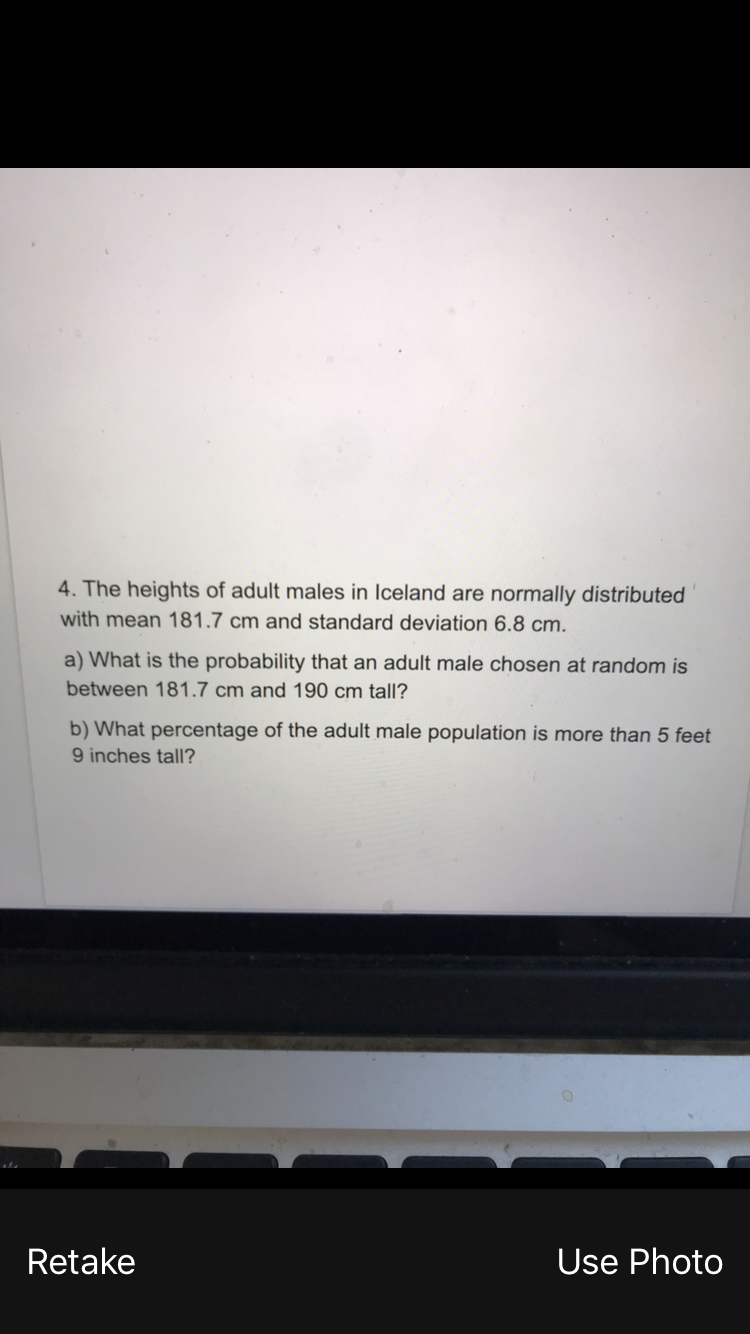# 4. The heights of adult males in Iceland are normally distributedwith mean 181.7 cm and standard deviation 6.8 cm.a) What is the probability that an adult male chosen at random isbetween 181.7 cm and 190 cm tall?b) What percentage of the adult male population is more than 5 feet9 inches tall?RetakeUse Photo

Question
297 viewshelp_outlineImage Transcriptionclose4. The heights of adult males in Iceland are normally distributed with mean 181.7 cm and standard deviation 6.8 cm. a) What is the probability that an adult male chosen at random is between 181.7 cm and 190 cm tall? b) What percentage of the adult male population is more than 5 feet 9 inches tall? Retake Use Photo fullscreen
check_circle

Step 1

Let X be the height of adult males in Iceland.  It is given that height of adult males follows Normla distribution with mean=181.7cm and standard deviation=6.8cm

To find probability the first step is to convert given x values into z score.The  z score formula is  shown on the board.

Step 2

a) Probability that an adult male chosen at random is between 181.7cm and 190cm tall is

Using Z score table to find the probability below z=1.22 and z=0

P(Z <1.22) = 0.8888 and  P(Z<0)= 0.5

So, P(Z<1.22) - P(Z<0) = 0.8888-0.5 = 0.3888

Hence, probabiity  that an adult male chosen at random is between 181.7cm and 190cm tall is 0.3888

Step 3

b) The percentage of adult male population is more than 5 feet and 9 inces tall

Convert 5feetand 9 inch into cm

1foot =12 inches

so, 5 feet =12*5 = 60inches

60+9inches=69inches

1Inch =2.54cm

So, 69inch = 69 *2....

### Want to see the full answer?

See Solution

#### Want to see this answer and more?

Solutions are written by subject experts who are available 24/7. Questions are typically answered within 1 hour.*

See Solution
*Response times may vary by subject and question.
Tagged in

### Other